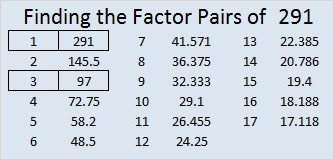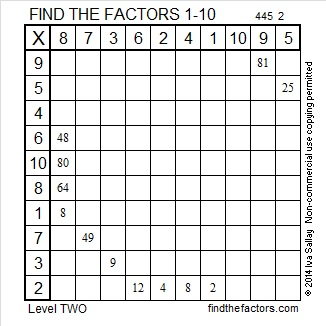# 291 and Level 2

• 291 is a composite number.
• Prime factorization: 291 = 3 x 97
• The exponents in the prime factorization are 1 and 1. Adding one to each and multiplying we get (1 + 1)(1 + 1) = 2 x 2 = 4. Therefore 291 has exactly 4 factors.
• Factors of 291: 1, 3, 97, 291
• Factor pairs: 291 = 1 x 291 or 3 x 97
• 291 has no square factors that allow its square root to be simplified. √291 ≈ 17.059Can you complete this multiplication table if you’re given just twelve clues?Print the puzzles or type the factors on this excel file: 10 Factors 2014-11-10